## 第三周：浅层神经网络(Shallow neural networks)

### 3.1 神经网络概述（Neural Network Overview）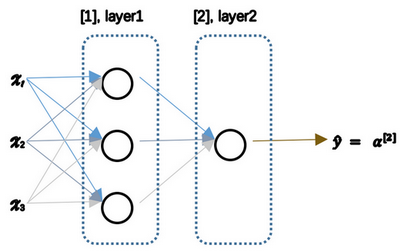### 3.2 神经网络的表示（Neural Network Representation）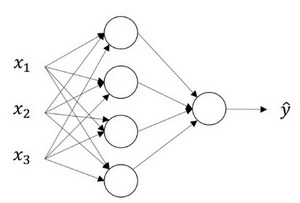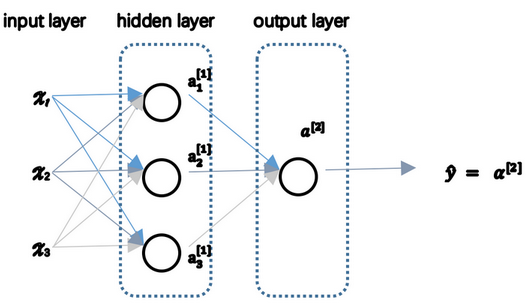图3.2.2

### 3.3 计算一个神经网络的输出（Computing a Neural Network's output）图3.3.1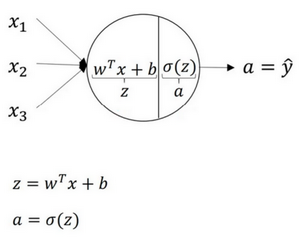图3.3.2

$z^{}_1 = w^{T}_1x + b^{}_1, a^{}_1 = \sigma(z^{}_1)$

$z^{}_2 = w^{T}_2x + b^{}_2, a^{}_2 = \sigma(z^{}_2)$

$z^{}_3 = w^{T}_3x + b^{}_3, a^{}_3 = \sigma(z^{}_3)$

$z^{}_4 = w^{T}_4x + b^{}_4, a^{}_4 = \sigma(z^{}_4)$

$a^{[n]}=\sigma(z^{[n]})$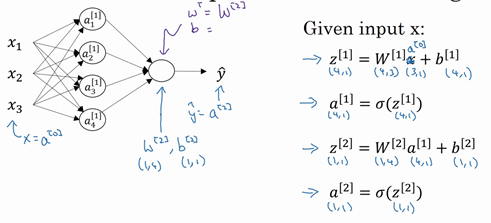图3.3.3

### 3.4 多样本向量化（Vectorizing across multiple examples）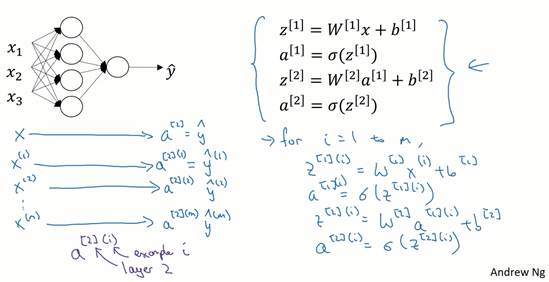图3.4.1

【注】：$a^{(i)}$$(i)$是指第$i$个训练样本而$$是指第二层。

$z^{(i)}=W^{(i)}x^{(i)}+b^{(i)}$

$a^{(i)}=\sigma(z^{(i)})$

$z^{(i)}=W^{(i)}a^{(i)}+b^{(i)}$

$a^{(i)}=\sigma(z^{(i)})$

### 3.5 向量化实现的解释（Justification for vectorized implementation）

$z^{(2)} = W^{}x^{(2)} + b^{}$

$z^{(3)} = W^{}x^{(3)} + b^{}$

### 3.6 激活函数（Activation functions）

sigmoid函数和tanh函数两者共同的缺点是，在$z$特别大或者特别小的情况下，导数的梯度或者函数的斜率会变得特别小，最后就会接近于0，导致降低梯度下降的速度。

$z$是负值时，这个函数的值不是等于0，而是轻微的倾斜，如图。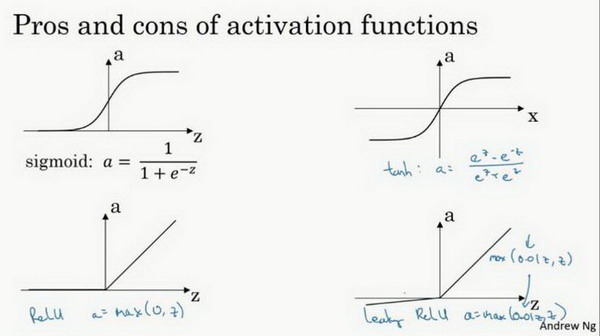图3.6.1

$z$ReLu的梯度一半都是0，但是，有足够的隐藏层使得z值大于0，所以对大多数的训练数据来说学习过程仍然可以很快。

sigmoid激活函数：除了输出层是一个二分类问题基本不会用它。

tanh激活函数：tanh是非常优秀的，几乎适合所有场合。

ReLu激活函数：最常用的默认函数，，如果不确定用哪个激活函数，就使用ReLu或者Leaky ReLu。公式3.23： $a = max( 0.01z,z)$ 为什么常数是0.01？当然，可以为学习算法选择不同的参数。

### 3.7 为什么需要非线性激活函数？（why need a nonlinear activation function?）

(2) $a^{} = z^{} = W^{}a^{}+ b^{}$ 将式子(1)代入式子(2)中，则： $a^{} = z^{} = W^{}(W^{}x + b^{}) + b^{}$

(3) $a^{} = z^{} = W^{}W^{}x + W^{}b^{} + b^{}$ 简化多项式得 $a^{} = z^{} = W^{'}x + b^{'}$ 如果你是用线性激活函数或者叫恒等激励函数，那么神经网络只是把输入线性组合再输出。

### 3.8 激活函数的导数（Derivatives of activation functions）

1）sigmoid activation function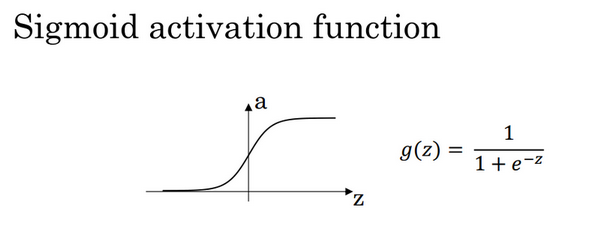图3.8.1

$z$ = 10或$z= -10$ ; $\frac{d}{dz}g(z)\approx0$

$z$= 0 , $\frac{d}{dz}g(z)\text{=g(z)(1-g(z))=}{1}/{4}$

2) Tanh activation function图3.8.2

$z$ = 10或$z= -10$ $\frac{d}{dz}g(z)\approx0$

$z$ = 0, $\frac{d}{dz}g(z)\text{=1-(0)=}1$

3）Rectified Linear Unit (ReLU)$g(z) =max (0,z)$

4）Leaky linear unit (Leaky ReLU)

ReLU类似

### 3.9 神经网络的梯度下降（Gradient descent for neural networks）

Cost function: 公式： $J(W^{},b^{},W^{},b^{}) = {\frac{1}{m}}\sum_{i=1}^mL(\hat{y}, y)$ loss function和之前做logistic回归完全一样。

$\hat{y}^{(i)},(i=1,2,…,m)$ 公式3.28： $dW^{} = \frac{dJ}{dW^{}},db^{} = \frac{dJ}{db^{}}$ 公式3.29： ${d}W^{} = \frac{{dJ}}{dW^{}},{d}b^{} = \frac{dJ}{db^{}}$

back propagation： 公式3.32： $dz^{} = A^{} - Y , Y = \begin{bmatrix}y^{} & y^{} & \cdots & y^{[m]}\\ \end{bmatrix}$ 公式3.33： $dW^{} = {\frac{1}{m}}dz^{}A^{T}$ 公式3.34： ${\rm d}b^{} = {\frac{1}{m}}np.sum({d}z^{},axis=1,keepdims=True)$ 公式3.35： $dz^{} = \underbrace{W^{T}{\rm d}z^{}}_{(n^{},m)}\quad*\underbrace{{g^{}}^{'}}_{activation \; function \; of \; hidden \; layer}*\quad\underbrace{(z^{})}_{(n^{},m)}$ 公式3.36：